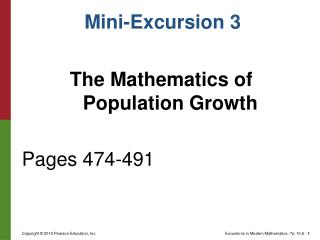DownloadDownload PresentationThe Mathematics of Population Growth Pages 474-491

The Mathematics of Population Growth Pages 474-491

Télécharger la présentationThe Mathematics of Population Growth Pages 474-491

- - - - - - - - - - - - - - - - - - - - - - - - - - - E N D - - - - - - - - - - - - - - - - - - - - - - - - - - -
Presentation Transcript

1. Mini-Excursion 3 The Mathematics of Population Growth Pages 474-491

2. Continuous vs. Discrete Growth • Population growth is a time-dependent process. • Discrete growth occurs when there are gaps in the growth times. • Continuous growth occurs without gaps between growth times.

3. Discrete Growth • During discrete population growth, there will be sudden changes in the population called a transition. • A mathematical model of population growth defines the transition rules in the form of an equation.

4. Discrete Growth • A population sequence is a sequence of numbers that represent the population levels at each transition time. The notation for a population sequence is: • is called the initial population. • The transition rule determines the population sequence.

5. Discrete Growth

6. Discrete Growth • A time-series graph shows the time of each transition on the horizontal axis and the size of the population on the vertical axis.

7. Discrete Growth

8. Linear Growth • For a lineargrowthmodel, each generation of the population increases (or decreases) by adding (or subtracting) fixed amount called the commondifference. For example: Initial population = 1, common difference = 4

9. Linear Growth • The time-series graph of a linear growth model makes a straight line.

10. Linear Growth • If the common difference is denoted d, the linear growth model is • This is called an arithmetic sequence.

11. Linear Growth • We can summarize the linear growth model with the formula:

12. Example • Problem 4 on page 488

13. Exponential Growth • For an exponential growthmodel, each generation of the population changes by multiplying a fixed amount called the commonratio. This is a geometric sequence. For example: Initial population = 1, common ratio = 5

14. Exponential Growth • The time-series graph of an exponential growth model makes an exponential curve.

15. Exponential Growth • If the common ratio is denoted r, the exponential growth model is • Or:

16. Example • A population grows according to an exponential growth model with: • Find the common ratio r • Find • Give an explicit formula for (this example is similar to assigned problems 11 and 12)

17. Logistic Growth • The linear growth model predicts unlimited growth as N gets larger if d is positive. • The exponential growth model predicts unlimited growth as N gets larger if r is larger than 1.

18. Logistic Growth • In reality, resources are limited and populations do not grow without limit. • The logistic growth model predicts resource limited growth as N gets larger.

19. Logistic Growth • In the logistic growth model represents the fraction of the population out of the total population allowed by the habitat called the carrying capacity of the habitat. • The value of will always be a number between 0 and 1.

20. Logistic Growth • page 483

21. Logistic Growth • Solution:

22. Logistic Growth • Solution:

23. Logistic Growth • Solution:

24. Logistic Growth • page 484

25. Logistic Growth • Solution

26. Logistic Growth • Solution

27. Logistic Growth • Solution

28. Logistic Growth • page 484-485

29. Logistic Growth • page 484-485

30. Logistic Growth • page 485

31. Logistic Growth • page 486

32. Logistic Growth • No pattern (chaos)